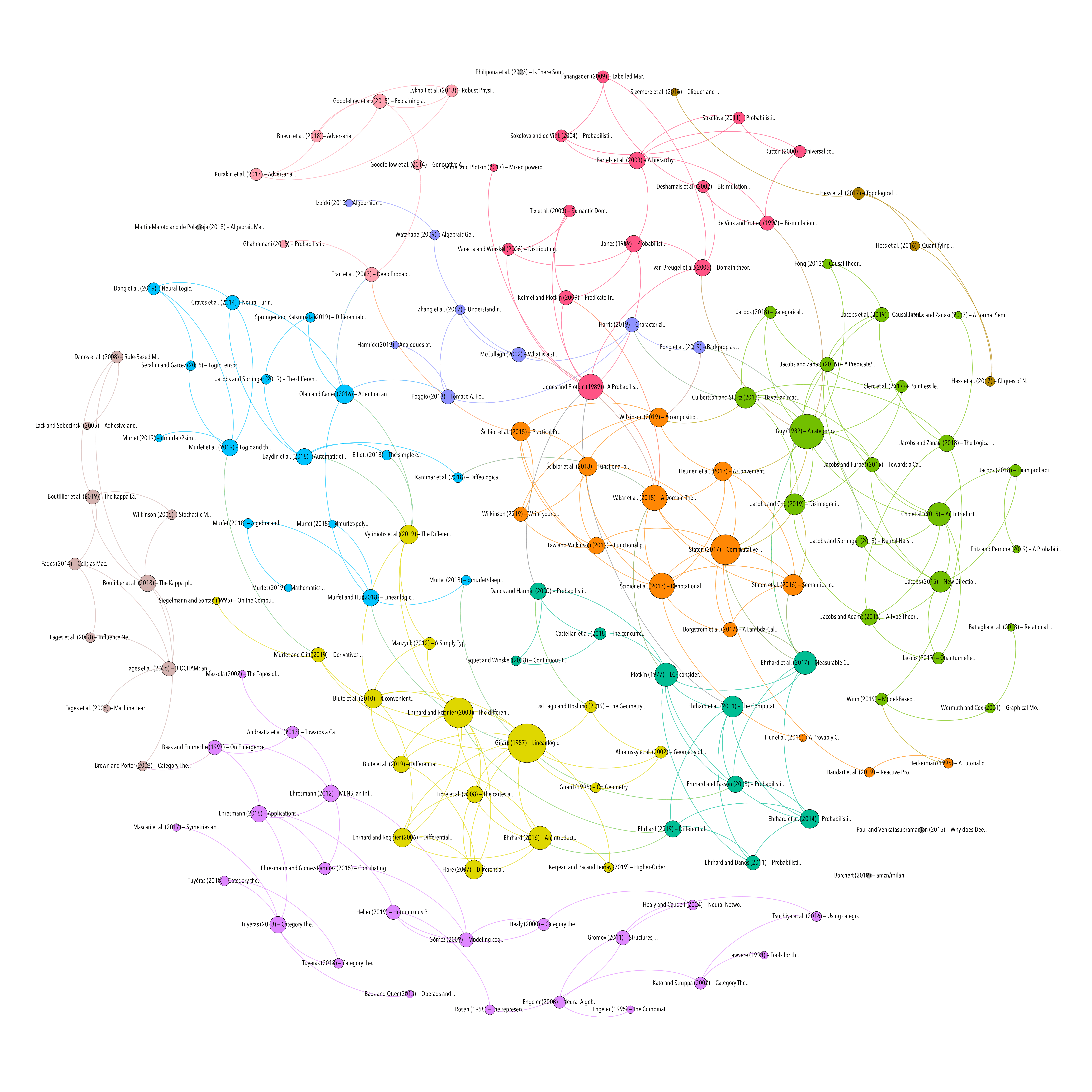# Full bibliography

## Characterizing the invariances of learning algorithms using category theory

Resource type
Author/contributor
Title
Characterizing the invariances of learning algorithms using category theory
Abstract
Many learning algorithms have invariances: when their training data is transformed in certain ways, the function they learn transforms in a predictable manner. Here we formalize this notion using concepts from the mathematical field of category theory. The invariances that a supervised learning algorithm possesses are formalized by categories of predictor and target spaces, whose morphisms represent the algorithm's invariances, and an index category whose morphisms represent permutations of the training examples. An invariant learning algorithm is a natural transformation between two functors from the product of these categories to the category of sets, representing training datasets and learned functions respectively. We illustrate the framework by characterizing and contrasting the invariances of linear regression and ridge regression.
Publication
arXiv:1905.02072 [cs, math, stat]
Date
2019-05-06
Accessed
2019-10-10T11:53:28Z
Library Catalog
Extra
arXiv: 1905.02072
Citation
Harris, K. D. (2019). Characterizing the invariances of learning algorithms using category theory. ArXiv:1905.02072 [Cs, Math, Stat]. Retrieved from http://arxiv.org/abs/1905.02072
MACHINE LEARNING
Methodology
Processing time: 0.02 secondsGraph of references (from Zotero to Gephi via Zotnet with this script)